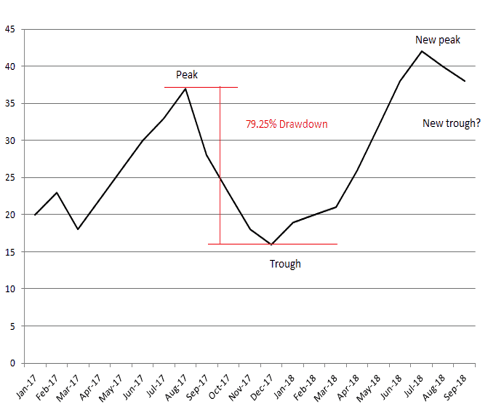# The 4 Best Parameters to Evaluate a Trading System

Developing a trading system is not a rocket science today. Anyone with an elementary knowledge of computer and technical analysis can build his/her own profitable system.

However, the question is whether that system would perform in a long run? What makes your system better than thousands of other systems that exist?

To answer these questions, we have identified the 4 important parameters that you should look into to Evaluate a Trading System. You may find these parameters in any standard backtesting report.

Also Read: Understanding Amibroker Backtest Report

## Parameter 1: Drawdown

Drawdown is the maximum capital you can lose through the system. Usually expressed in %, it specifies the maximum peak to trough decline in the equity curve. So, if your capital is 10000, and the backtesting shows that the capital can fall up to 9000 due to losses realized, then your max drawdown is 1000 or 10%.Calculation:

Max Drawdown = Peak Capital – Lowest Capital

Max Drawdown % = (Peak Capital – Lowest Capital)/Peak Captal * 100

Check out this article to see how to calculate drawdown in the excel sheet.

“Lower the max drawdown %, better is the system”

## Parameter 2: Average Risk Reward Ratio

This is the ratio of average loss and average profit based on the backtesting report. For example – if the average loss the system encountered over a period of time is 500, and the average profit is 1000, then the risk to reward ratio is 500:1000 or 1:2.

The risk-reward ratio is correlated with the success rate of the system. If your risk-reward ratio is 1:2 or 0.5, then you will be profitable if you win 33% of your trades. Or in other words, even if your 67% of trades end up in a loss, your net portfolio will still be in green if the risk-reward ratio is 1:2. If you are able to lower the risk-reward ratio further, then the breakeven success rate also will reduce.

Calculation:

Average Risk-Reward Ratio = Average Loss/Average Profit

Check out the below article to understand the significance of the risk-reward ratio:

“Lower the risk-reward ratio, better is the system”

## Parameter 3: Maximum Losing Streak

It’s the maximum losses that can happen in a row. Though this parameter has nothing to do with the overall performance of the system, it is an important phycological factor that determines if you can really trade based on this system. Traders generally don’t prefer any system that has a maximum losing streak of less than 5.

The below table illustrates the maximum losing streak:“Maximum losing streak should not go beyond 5”

## Parameter 4: Sharpe Ratio

In our view, the Sharpe ratio is the best method to evaluate a trading system. It measures the average returns adjusting for the risks that allow systems of different risk profiles to be compared against each other. Sharpe ratio is calculated by taking the difference between the overall return and the return on a risk-free investment. That difference is then divided by the standard deviation.

Sharpe ratio essentially provides the below two information:

1. How the system is faring against a risk-free return rate? Practically none of the investments are risk-free but for calculation purposes, treasury bond yield or bank FD interest rates are considered for this.
2. How volatile is the system? The volatility is estimated using the standard deviation of returns.

Calculation:

Sharpe Ratio = (Rp – Rf)/σp

where

Rp = System Return (in %)

Rf = Risk Free return rate (in %)

σp = Standard Deviation (in %)

“Ignore the systems that have Sharpe ratio below 2, higher the better”

All these parameters are readily available in the backtest report of Amibroker.You can access the sample backtest report from this link.

The backtest was done on RSI-DEMA intraday system which is part of our professional trading system development course.

Apart from these four, you can use several other parameters to evaluate a trading system. CAGR, Expectancy, K-Ratio, Ulcer Index, etc are some of the notable ones. However, filter out the inefficient systems based on max drawdown, risk-reward ratio, Sharpe ratio, and max losing streak first, and then look at these other parameters.

Let us know in the comments section if you have any questions.